## Linear Transformation

An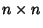Matrixis a linear transformation (linear Map) Iff, for every pair of-Vectorsandand every Scalar,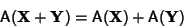(1)

and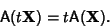(2)

Consider the 2-D transformation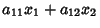(3)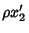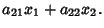(4)

Rescale by definingand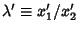, then the above equations become(5)

whereand,,and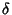are defined in terms of the old constants. Solving for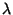gives(6)

so the transformation is One-to-One. To find the Fixed Points of the transformation, setto obtain(7)

This gives two fixed points which may be distinct or coincident. The fixed points are classified as follows.

 variables typeHyperbolic Fixed PointElliptic Fixed PointParabolic Fixed Point

See also Elliptic Fixed Point (Map), Hyperbolic Fixed Point (Map), Involuntary, Linear Operator, Parabolic Fixed Point

References

Woods, F. S. Higher Geometry: An Introduction to Advanced Methods in Analytic Geometry. New York: Dover, pp. 13-15, 1961.